# Ok = (6) Let n be a positive integer. For every integer k, define the 2...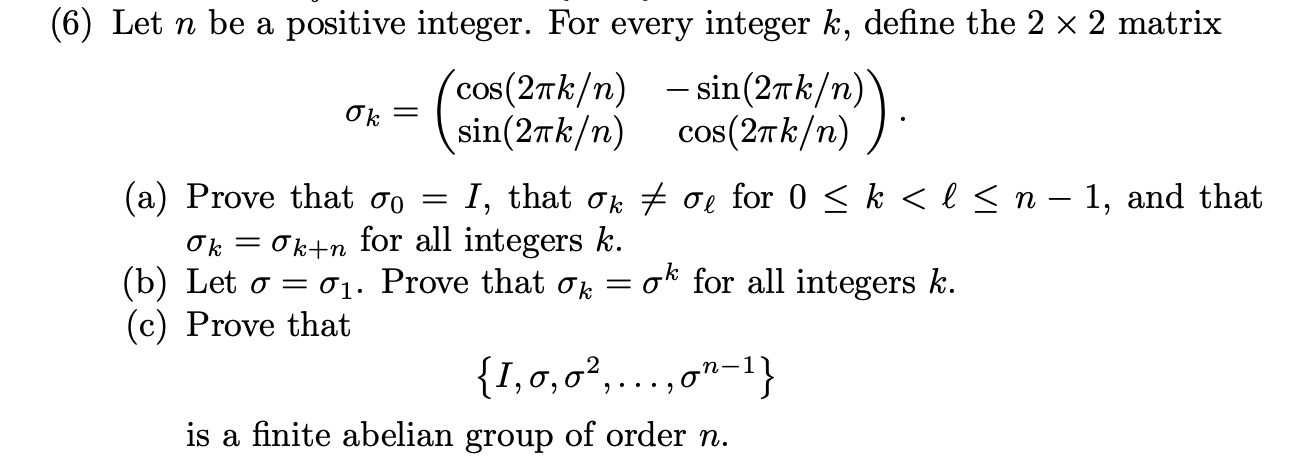Ok = (6) Let n be a positive integer. For every integer k, define the 2 x 2 matrix cos(27k/n) - sin(2nk/n) sin(2tk/n) cos(27 k/n) (a) Prove that go = I, that ok + oe for 0 < k < l< n - 1, and that Ok = Okun for all integers k. (b) Let o = 01. Prove that ok ok for all integers k. (c) Prove that {1,0,0%,...,ON-1} is a finite abelian group of order n.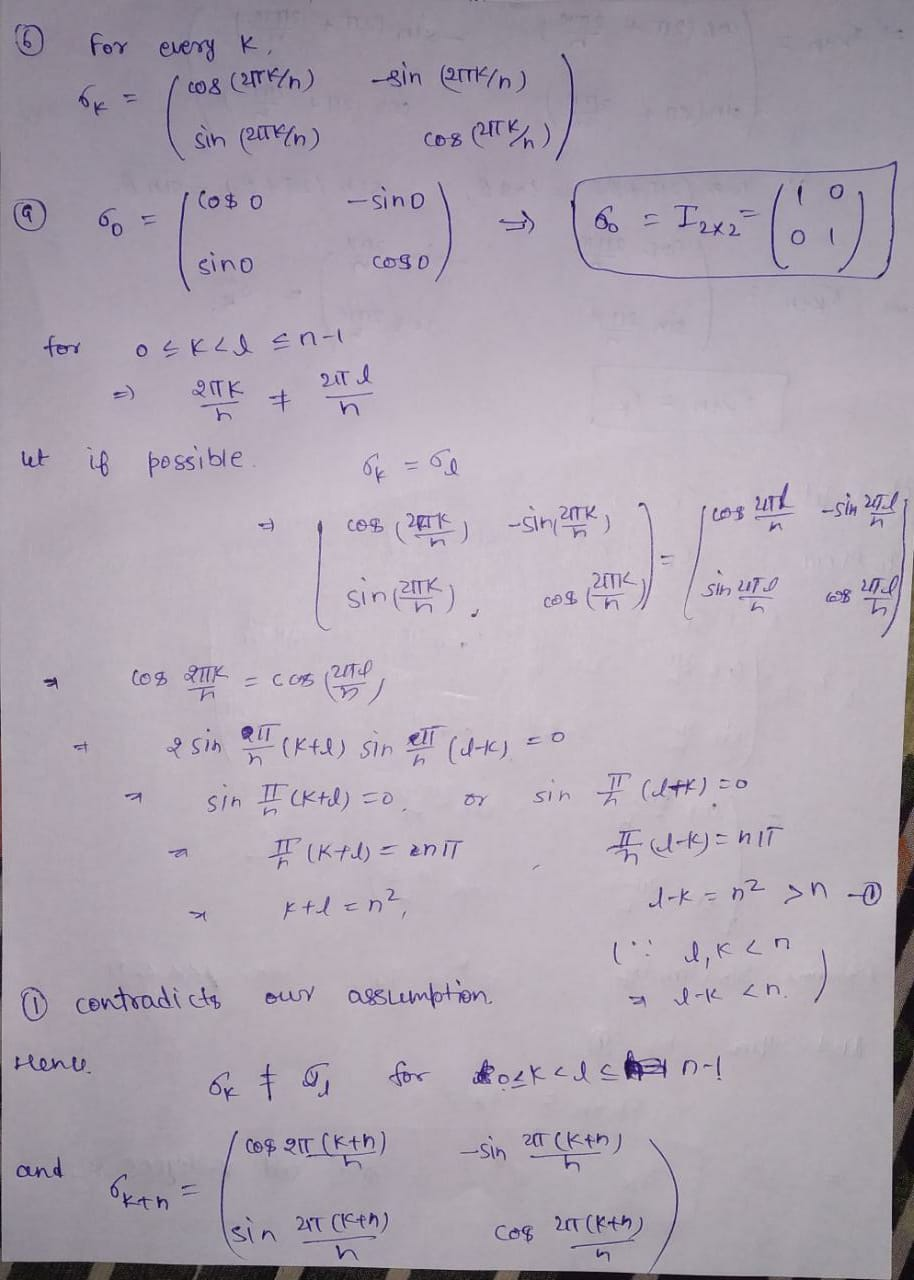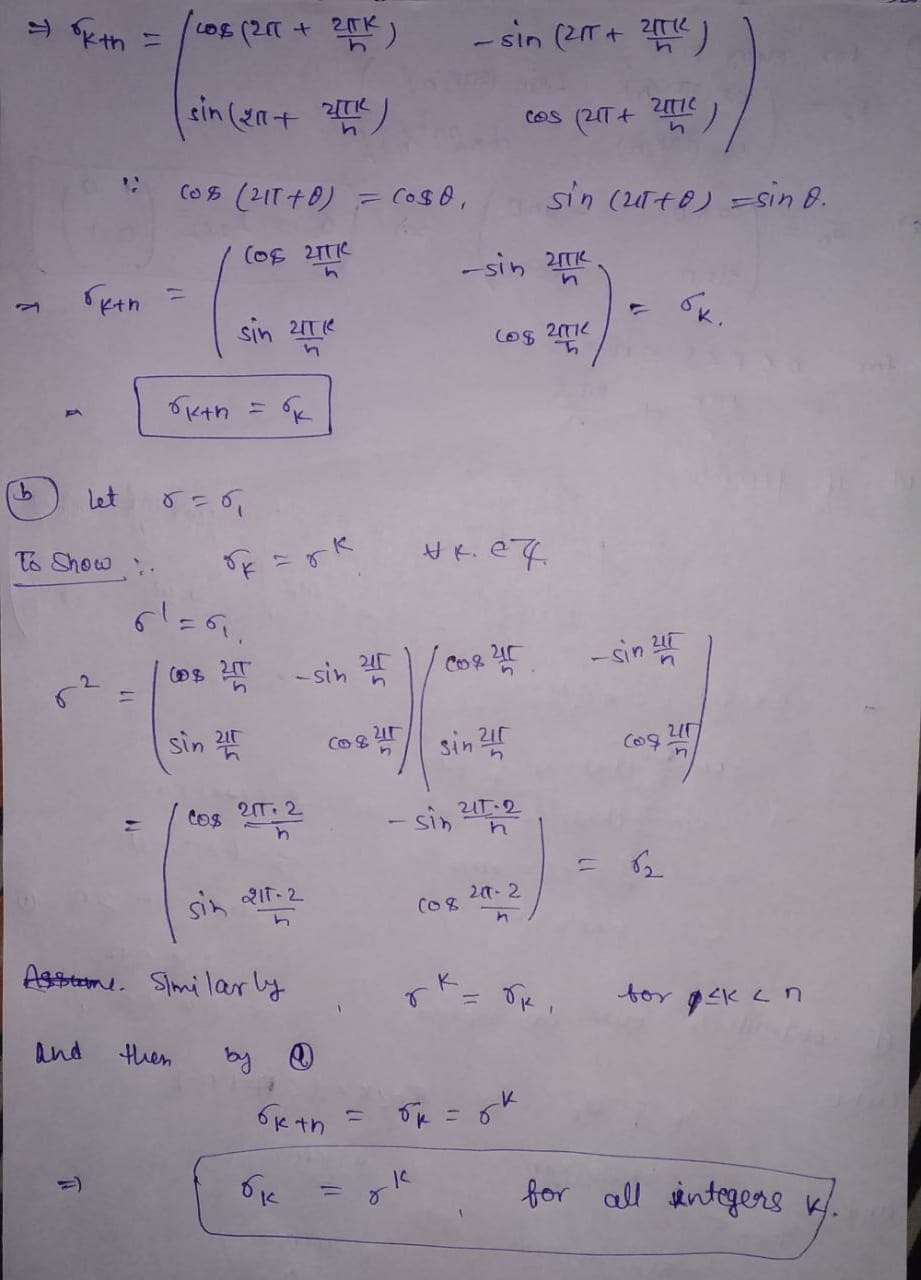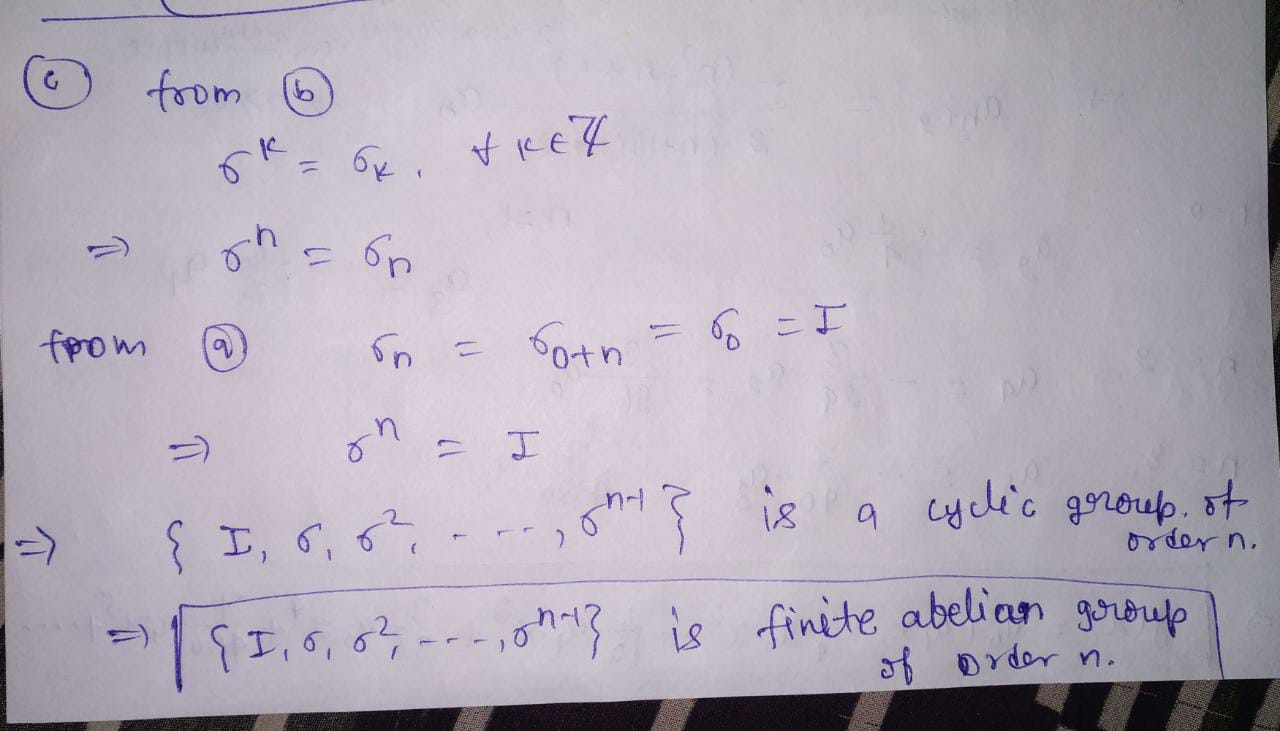##### Add Answer of: Ok = (6) Let n be a positive integer. For every integer k, define the 2...
Similar Homework Help Questions
• ### Let G be a finite group, prove that for every a in G there exists a positive integer n such that ...

let G be a finite group, prove that for every a in G there exists a positive integer n such that an=e, the identity.

• ### (a) Let A be a Hermitian matrix. DEFINE: A is positive definite. (b) Let A be an n × n Hermitian ...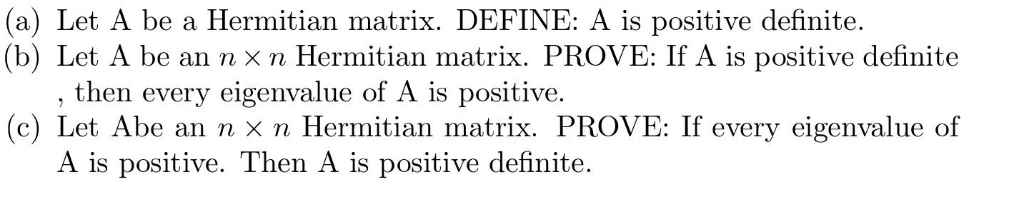(a) Let A be a Hermitian matrix. DEFINE: A is positive definite. (b) Let A be an n × n Hermitian matrix. PROVE: If A is positive definite the n every eigenvalue of A is positiv e. (c) Let Abe an n X n Hermitian matrix. PROVE: If every eigenvalue of A is positive. Then A is positive definite. (a) Let A be a Hermitian matrix. DEFINE: A is positive definite. (b) Let A be an n × n Hermitian...

• ### Prove: Let k be a positive integer, and set n :=2k-1(2k – 1). Then (2k+1 –...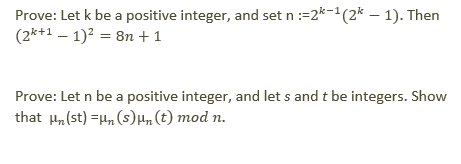Prove: Let k be a positive integer, and set n :=2k-1(2k – 1). Then (2k+1 – 1)2 = 8n +1 Prove: Let n be a positive integer, and let s and t be integers. Show that Hire (st) = n(s) in (t) mod n.

• ### 4) Let D be the set of all finite subsets of positive integers. Define a function...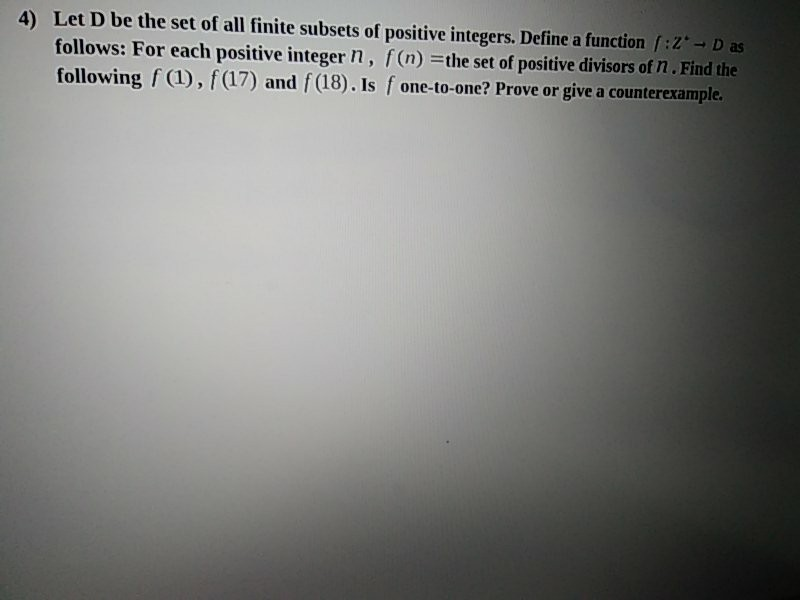4) Let D be the set of all finite subsets of positive integers. Define a function (:2 - D as follows: For each positive integer n, f(n) =the set of positive divisors of n. Find the following f (1), f(17) and f(18). Is f one-to-one? Prove or give a counterexample.

• ### LIFESAVER, Show that if G is an abelian group, and m is a positive integer, then Gm is a...Let G be an abelian group with identityelement e. For every positiveinteger m, let Gm and Gm be subsets of Gdefined as follows:Gm = {xm | xG} and Gm={xG | xm = e}.In other words, Gm consists of all m-th powersof elements of G, and Gm consists all elements ofG whose m-th power equals the identity.1. Show that if G is an abelian group, and m is a positive integer,then Gm is a subgroup of G.(You may assume that if...

• ### QUESTION C. (a) Let k be a field and let n be a positive integer. Define what is meant by a monomial ideal in k[x,.....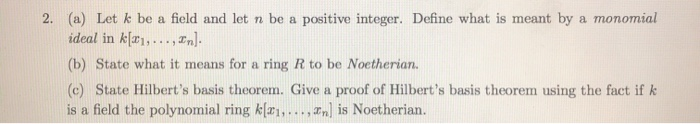QUESTION C. (a) Let k be a field and let n be a positive integer. Define what is meant by a monomial ideal in k[x,...,zn]. 2. (b) State what it means for a ring R to be Noetherian. (c) State Hilbert's basis theorem. Give a proof of Hilbert's basis theorem using the fact if k is a field the polynomial ring kli,..., In] is Noetherian. 1S (a) Let k be a field and let n be a positive integer. Define...

• ### Theorem: For every integer n, if x and y are positive integers with max(x, y) = n, then x = y

Theorem: For every integer n, if x and y are positive integers with max(x, y) = n, then x = y.Basic Step: Suppose that n = 1. If max(x, y) = 1 and x and y are positive integers, we have x = 1 and y = 1.Inductive Step: Let k be a positive integer. Assume that whenever max(x, y) = k and x and y are positive integers, then x = y.Now let max(x, y) = k + 1,...

• ### Let a, b, c, and d be integers, and let n be a positive integer

Let a, b, c, and d be integers, and let n be a positive integer. Prove that if a is congruent to c mod n and b is congruent to d mod n, then (a-b) is congruent to (c-d) mod n

• ### 2. Let n be a positive integer. Denote the number of positive integers less than n and rela- tive...number thoery just need 2 answered 2. Let n be a positive integer. Denote the number of positive integers less than n and rela- tively prime to n by p(n). Let a, b be positive integers such that ged(a,n) god(b,n)-1 Consider the set s, = {(a), (ba), (ba), ) (see Prollern 1). Let s-A]. Show that slp(n). 1. Let a, b, c, and n be positive integers such that gcd(a, n) = gcd(b, n) = gcd(c, n) = 1 If...

• ### (2) For an integer n, let Z/nZ denote the set of equivalence classes [k) tez: k -é is divisible by n (a) Prove that the set Z/nZ has n elements. (b) Find a minimal set of representatives for these...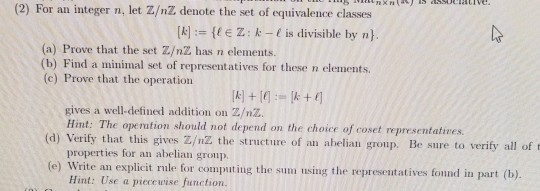(2) For an integer n, let Z/nZ denote the set of equivalence classes [k) tez: k -é is divisible by n (a) Prove that the set Z/nZ has n elements. (b) Find a minimal set of representatives for these n elements. (c) Prove that the operation gives a well-defined addition on Z/nZ Hint: The operution should not depend on the choice of coset representatives Verify that this gives Z/n2 the structure of an ahelian group. Be sure to verify all...

Free Homework App